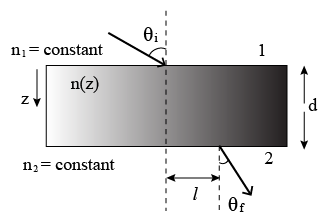Instructions

For the following questions answer them individually

Question 11

Question 12

Question 13

# A transparent slab of thickness d has a refractive index n(z) that increases with z. Here z is the vertical distance inside the slab, measured from the top. The slab is placed between two media with uniform refractive indices $$n_1$$ and $$n_2(> n_1)$$, as shown in the figure. A ray of light is incident with angle $$\theta_i$$ from medium 1 and emerges in medium 2 with refraction angle $$\theta_f$$, with a lateral displacement l.Which of the following statement(s) is(are) true?

Instructions

The answer to each question is a SINGLE DIGIT INTEGER ranging from 0 to 9, both inclusive.

Question 14

Backspace
789
456
123
0.-
Clear All
Question 15

Backspace
789
456
123
0.-
Clear All
Question 16

Backspace
789
456
123
0.-
Clear All
Question 17

Backspace
789
456
123
0.-
Clear All
Question 18

# Two inductors $$L_1$$(inductance 1 mH, internal resistance 3 Ω) and $$L_2$$ (inductance 2 mH, internal resistance 4 Ω), and a resistor R (resistance 12 Ω) are all connected in parallel across a 5 V battery. The circuit is switched on at time ¢=0. The ratio of the maximum to the minimum current $$\left(\frac{I_{max}}{I_{min}}\right)$$ drawn from the battery is

Backspace
789
456
123
0.-
Clear All
Instructions

For the following questions answer them individually

Question 19

Question 20

OR